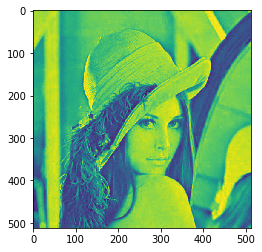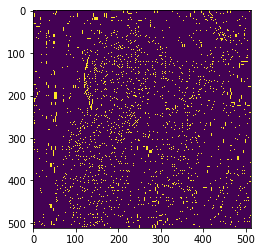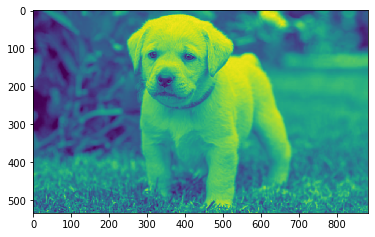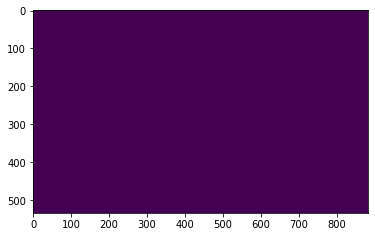Related Articles

# Mahotas – Regional Maxima of Image

• Last Updated : 12 May, 2021

In this article we will see how we can get regional maxima of image in mahotas. Regional maxima is a stricter criterion than the local maxima as it takes the whole object into account and not just the neighborhood. Maxima are connected components of pixels with a constant intensity value, surrounded by pixels with a lower value.

In this tutorial we will use “lena” image, below is the command to load it.

`mahotas.demos.load('lena')`

Below is the lena imageIn order to do this we will use mahotas.regmax method
Syntax : mahotas.regmax(img)
Argument : It takes image object as argument
Return : It returns image object

Note : Input image should be filtered or should be loaded as grey

In order to filter the image we will take the image object which is numpy.ndarray and filter it with the help of indexing, below is the command to do this

`image = image[:, :, 0]`

Below is the implementation

## Python3

 `# importing required libraries``import` `mahotas``import` `mahotas.demos``from` `pylab ``import` `gray, imshow, show``import` `numpy as np``import` `matplotlib.pyplot as plt``  ` `# loading image``img ``=` `mahotas.demos.load(``'lena'``)``  ` `# filtering image``img ``=` `img.``max``(``2``)` `print``(``"Image"``)``  ` `# showing image``imshow(img)``show()``# finding regional maxima``new_img ``=` `mahotas.regmax(img)`` `  `# showing image``print``(``"Regional Maxima"``)``imshow(new_img)``show()`

Output :

```Image
````Regional Maxima`Another example

## Python3

 `# importing required libraries``import` `mahotas``import` `numpy as np``from` `pylab ``import` `gray, imshow, show``import` `os``import` `matplotlib.pyplot as plt`` ` `# loading image``img ``=` `mahotas.imread(``'dog_image.png'``)`  `# filtering image``img ``=` `img[:, :, ``0``]``  ` `print``(``"Image"``)``  ` `# showing image``imshow(img)``show()` `# finding regional maxima``new_img ``=` `mahotas.regmax(img)`` `  `# showing image``print``(``"Regional Maxima"``)``imshow(new_img)``show()`

Output :

`Image``Regional Maxima`Attention geek! Strengthen your foundations with the Python Programming Foundation Course and learn the basics.

To begin with, your interview preparations Enhance your Data Structures concepts with the Python DS Course. And to begin with your Machine Learning Journey, join the Machine Learning – Basic Level Course

My Personal Notes arrow_drop_up